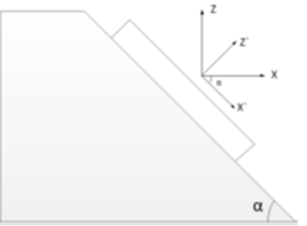top of page# Piezoelectric Material on Sloped Plane

The piezoelectric effect is the linear electromechanical interaction between the mechanical and electrical state in crystalline materials. The piezoelectric constant is rigorously defined using tensor analysis. Thus, the orientation of the piezo crystal material is very important to the piezoelectric analysis. Here we present an interesting case wherein the piezo material is on the angled walls (Fig 1). To accurately simulate this device, we need to model the piezo material property with the local angle information and anisotropic piezoelectric material property for each angled structure.Fig 1: Cross section of PZT thin film

The piezoelectric material is sandwiched between two thin metal electrodes either Pt or Ti layers. The piezoelectric layer must be divided into several parts because the centre pit changes with the orientation of PZT material on the slope (Fig 2). In the interest of defining the material orientation and speed-up the simulation, we can simulate half and extract the result with symmetry boundary condition.Fig 2 : a) Full 3D model of the device, b) Cross-section view of the device with symmetric BC

Static, Frequency and Dynamic analysis can be performed by defining the piezoelectric material with their strain coefficient matrix on all top layer entities (Fig 3). It is important to note that the local material orientations are not the same as global coordinate.

To define a rectangular Cartesian system by the two points a, b, point a must lie on the X’-axis, and point b must lie on the X’-Y’ plane. Although not necessary, it is intuitive to select point b such that it is on or near the local Y’-axis.

If we consider one of the piezoelectric material on the left slope, the local coordinate is the rotation along Y-axis of global coordinate. So, the point b is any point on the Y – axis and the point a is any multiple of 1, 0, -tanα.  For the piezoelectric material at the corner, the material orientation is between general orientation and local slope orientation, so the point b is the same and the point a is any multiple of (1, 0, -tanα/2).Fig 3: Defining strain matrixFig 4: Local slope coordinate

Fixed Boundary Conditions are applied, and floating voltages are defined to the two electrodes and a pressure of 1bar is applied on the bottom surface of the device. We find the maximum displacement (Fig 5), Von Mises Stress (Fig 6) and the potential generated (Fig 7).Fig 5 : DisplacementFig 6: Potential DistributionFig 7: Potential Generated

bottom of page